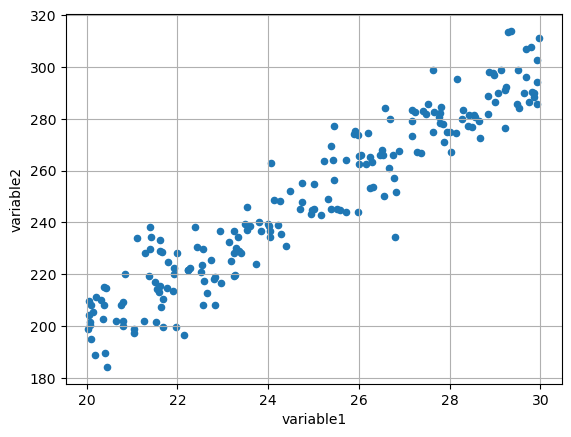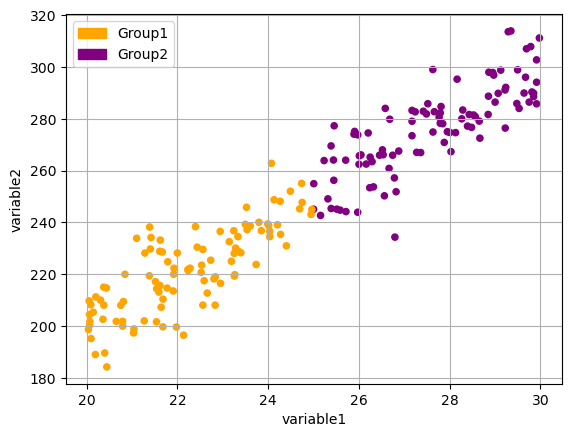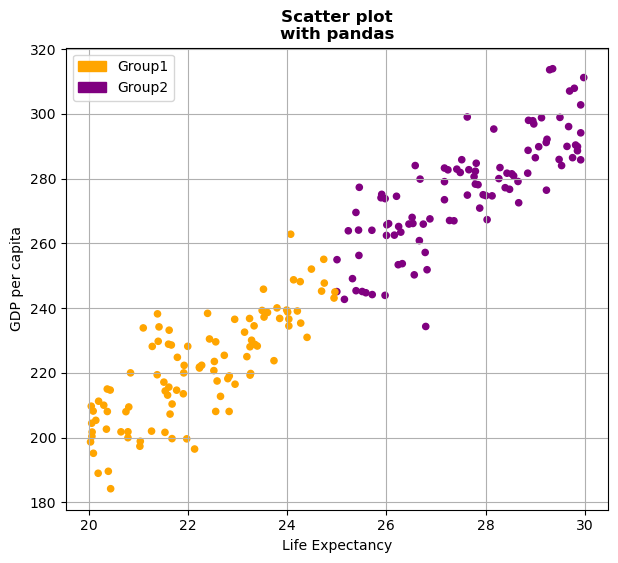A scatter plot is a graphical representation of data points in a dataset, where individual data points are plotted on a two-dimensional coordinate system. This type of plot allows us to visualize the relationship between two variables by showing how they are distributed across the plot.
Pandas, a powerful data manipulation library in Python, allow us to create easily scatter plots: check this introduction to scatter plots with pandas. In this post, we will explore how to leverage `Pandas` to customize scatter plots with a grouping variable.

## Libraries

Pandas is a popular open-source Python library used for data manipulation and analysis. It provides data structures and functions that make working with structured data, such as tabular data (like `Excel` spreadsheets or `SQL` tables), easy and intuitive.

To install Pandas, you can use the following command in your command-line interface (such as `Terminal` or `Command Prompt`):

`pip install pandas`

Matplotlib functionalities have been integrated into the pandas library, facilitating their use with `dataframes` and `series`. For this reason, you might also need to import the matplotlib library when building charts with Pandas.

This also means that they use the same functions, and if you already know Matplotlib, you'll have no trouble learning plots with Pandas.

``````import pandas as pd
import numpy as np # data generation
import matplotlib.pyplot as plt``````

## Dataset

In order to create graphics with Pandas, we need to use pandas objects: `Dataframes` and `Series`. A dataframe can be seen as an `Excel` table, and a series as a `column` in that table. This means that we must systematically convert our data into a format used by pandas.

We generate 3 variables: 2 quantitative using `np.random.uniform()` and `np.random.normal()` functions and one qualitative, whose values depend on the values of the first qualitative variable.

``````# Generate a sample of 100 observations
sample_size = 200
first_variable = np.random.uniform(30, 20, sample_size)
second_variable = first_variable * 10 + np.random.normal(0, 10, sample_size)
categorical_variable = ['Group1' if i < 25 else 'Group2' for i in first_variable]

# Put the data into a pandas df
df = pd.DataFrame({'variable1': first_variable,
'variable2': second_variable,
'categorical_variable': categorical_variable}
)``````

## Basic scatter plot

Once we've opened our dataset, we'll now create the graph. The following displays the relation between the life expectancy and the gdp/capita using the `scatter()` function. This is probably one of the shortest ways to display a scatter plot in Python.

``````df.plot.scatter('variable1', # x-axis
'variable2', # y-axis
grid=True, # Add a grid in the background
)
plt.show()``````## Scatter plot with grouping

From a list comprehension, we create a list of colors according to the group of in the variable `categorical_variable`. Once this list is created, we just have to add the `c=colors` argument when calling the `scatter()` function.

To display the legend with the associated colors for each group in your scatter plot, you can create a legend with the handles and labels for each group. You can do this by manually creating a legend with `Patch` objects from `matplotlib.patches`.

``````# Get color for each data point
colors = {'Group1': 'orange', 'Group2': 'purple'}
color_list = [colors[group] for group in df['categorical_variable']]

# Create a scatter plot with color-coding based on 'categorical_variable'
ax = df.plot.scatter('variable1',
'variable2',
c=color_list,
grid=True)

# Create legend handles, labels for each group and add legend to the plot
import matplotlib.patches as mpatches
legend_handles = [
mpatches.Patch(color=colors['Group1'], label='Group1'),
mpatches.Patch(color=colors['Group2'], label='Group2'), # add as many as needed
]
ax.legend(handles=legend_handles,
loc='upper left')

plt.show()``````## Format layout

Here we'll see how to add some labels and the change the size of the figure.

• add labels: `set_title()` and `set_xlabel()` functions
• change the figure size: add the `figsize(width,height)` argument when using the `scatter()` function
``````# Get color for each data point
colors = {'Group1': 'orange', 'Group2': 'purple'}
color_list = [colors[group] for group in df['categorical_variable']]

# Create a scatter plot with color-coding based on 'categorical_variable'
ax = df.plot.scatter('variable1',
'variable2',
c=color_list,
grid=True,
figsize=(7,6))

# Create legend handles, labels for each group and add legend to the plot
import matplotlib.patches as mpatches
legend_handles = [
mpatches.Patch(color=colors['Group1'], label='Group1'),
mpatches.Patch(color=colors['Group2'], label='Group2'), # add as many as needed
]
ax.legend(handles=legend_handles,
loc='upper left')

# Add title and labels ('\n' allow us to jump rows)
ax.set_title('Scatter plot\nwith pandas',
weight='bold')
ax.set_xlabel('Life Expectancy')
ax.set_ylabel('GDP per capita')

plt.show()``````## Going further

This post explains how to create a scatter plot with grouping built with pandas.

For more examples of how to create or customize your plots with Pandas, see the pandas section. You may also be interested in how to customize your scatter plot.

## Contact & Edit

👋 This document is a work by Yan Holtz. You can contribute on github, send me a feedback on twitter or subscribe to the newsletter to know when new examples are published! 🔥